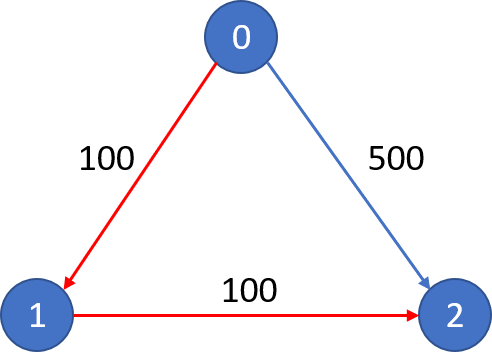##### Welcome to Subscribe On Youtube

Formatted question description: https://leetcode.ca/all/787.html

# 787. Cheapest Flights Within K Stops (Medium)

There are n cities connected by m flights. Each flight starts from city u and arrives at v with a price w.

Now given all the cities and flights, together with starting city src and the destination dst, your task is to find the cheapest price from src to dst with up to k stops. If there is no such route, output -1.

Example 1:
Input:
n = 3, edges = [[0,1,100],[1,2,100],[0,2,500]]
src = 0, dst = 2, k = 1
Output: 200
Explanation:
The graph looks like this:The cheapest price from city 0 to city 2 with at most 1 stop costs 200, as marked red in the picture.
Example 2:
Input:
n = 3, edges = [[0,1,100],[1,2,100],[0,2,500]]
src = 0, dst = 2, k = 0
Output: 500
Explanation:
The graph looks like this:The cheapest price from city 0 to city 2 with at most 0 stop costs 500, as marked blue in the picture.


Constraints:

• The number of nodes n will be in range [1, 100], with nodes labeled from 0 to n - 1.
• The size of flights will be in range [0, n * (n - 1) / 2].
• The format of each flight will be (src, dst, price).
• The price of each flight will be in the range [1, 10000].
• k is in the range of [0, n - 1].
• There will not be any duplicated flights or self cycles.

Related Topics:

Similar Questions:

## Solution 1. Bellman ford

// OJ: https://leetcode.com/problems/cheapest-flights-within-k-stops/
// Time: O(K * (N+ E))
// Space: O(N)
class Solution {
public:
int findCheapestPrice(int n, vector<vector<int>>& A, int src, int dst, int K) {
vector<int> dist(n, 1e8);
dist[src] = 0;
for (int i = 0; i <= K; ++i) {
vector<int> tmp = dist;
for (auto &e : A) {
int u = e, v = e, w = e;
dist[v] = min(dist[v], tmp[u] + w);
}
}
return dist[dst] == 1e8 ? -1 : dist[dst];
}
};

• class Solution {
public int findCheapestPrice(int n, int[][] flights, int src, int dst, int K) {
int[] prices = new int[n];
for (int i = 0; i < n; i++)
prices[i] = Integer.MAX_VALUE;
prices[src] = 0;
Map<Integer, List<int[]>> flightsMap = new HashMap<Integer, List<int[]>>();
for (int[] flight : flights) {
int flightSrc = flight, flightDst = flight, flightPrice = flight;
List<int[]> flightsList = flightsMap.getOrDefault(flightSrc, new ArrayList<int[]>());
flightsMap.put(flightSrc, flightsList);
}
queue.offer(src);
int remain = K;
while (remain >= 0 && !queue.isEmpty()) {
int[] tempPrices = new int[n];
for (int i = 0; i < n; i++)
tempPrices[i] = prices[i];
int size = queue.size();
for (int i = 0; i < size; i++) {
int curSrc = queue.poll();
int curPrice = prices[curSrc];
List<int[]> curFlightsList = flightsMap.getOrDefault(curSrc, new ArrayList<int[]>());
for (int[] flight : curFlightsList) {
int flightDst = flight, flightPrice = flight;
int newPrice = curPrice + flightPrice;
if (newPrice < tempPrices[flightDst]) {
tempPrices[flightDst] = newPrice;
queue.offer(flightDst);
}
}
}
for (int i = 0; i < n; i++)
prices[i] = tempPrices[i];
remain--;
}
int totalPrice = prices[dst];
}
}

############

class Solution {
private static final int INF = 0x3f3f3f3f;

public int findCheapestPrice(int n, int[][] flights, int src, int dst, int k) {
int[] dist = new int[n];
int[] backup = new int[n];
Arrays.fill(dist, INF);
dist[src] = 0;
for (int i = 0; i < k + 1; ++i) {
System.arraycopy(dist, 0, backup, 0, n);
for (int[] e : flights) {
int f = e, t = e, p = e;
dist[t] = Math.min(dist[t], backup[f] + p);
}
}
return dist[dst] == INF ? -1 : dist[dst];
}
}

• // OJ: https://leetcode.com/problems/cheapest-flights-within-k-stops/
// Time: O(K * (N+ E))
// Space: O(N)
class Solution {
public:
int findCheapestPrice(int n, vector<vector<int>>& A, int src, int dst, int K) {
vector<int> dist(n, 1e8);
dist[src] = 0;
for (int i = 0; i <= K; ++i) {
auto tmp = dist;
for (auto &e : A) {
int u = e, v = e, w = e;
dist[v] = min(dist[v], tmp[u] + w);
}
}
return dist[dst] == 1e8 ? -1 : dist[dst];
}
};

• class Solution:
def findCheapestPrice(
self, n: int, flights: List[List[int]], src: int, dst: int, k: int
) -> int:
INF = 0x3F3F3F3F
dist = [INF] * n
dist[src] = 0
for _ in range(k + 1):
backup = dist.copy()
for f, t, p in flights:
dist[t] = min(dist[t], backup[f] + p)
return -1 if dist[dst] == INF else dist[dst]

############

class Solution(object):
def findCheapestPrice(self, n, flights, src, dst, K):
"""
:type n: int
:type flights: List[List[int]]
:type src: int
:type dst: int
:type K: int
:rtype: int
"""
graph = collections.defaultdict(dict)
for u, v, e in flights:
graph[u][v] = e
visited =  * n
ans = [float('inf')]
self.dfs(graph, src, dst, K + 1, 0, visited, ans)
return -1 if ans == float('inf') else ans

def dfs(self, graph, src, dst, k, cost, visited, ans):
if src == dst:
ans = cost
return
if k == 0:
return
for v, e in graph[src].items():
if visited[v]: continue
if cost + e > ans: continue
visited[v] = 1
self.dfs(graph, v, dst, k - 1, cost + e, visited, ans)
visited[v] = 0

• func findCheapestPrice(n int, flights [][]int, src int, dst int, k int) int {
const inf = 0x3f3f3f3f
dist := make([]int, n)
backup := make([]int, n)
for i := range dist {
dist[i] = inf
}
dist[src] = 0
for i := 0; i < k+1; i++ {
copy(backup, dist)
for _, e := range flights {
f, t, p := e, e, e
dist[t] = min(dist[t], backup[f]+p)
}
}
if dist[dst] == inf {
return -1
}
return dist[dst]
}

func min(a, b int) int {
if a < b {
return a
}
return b
}